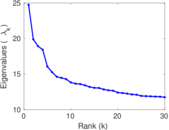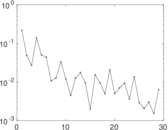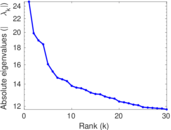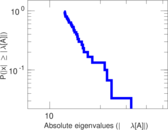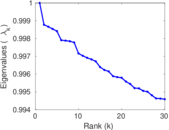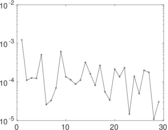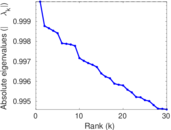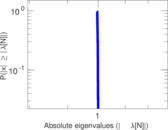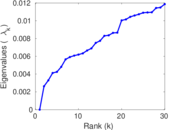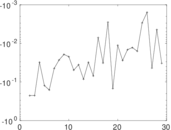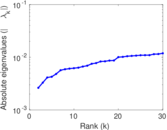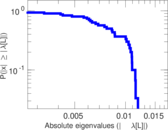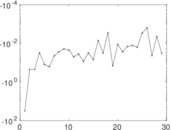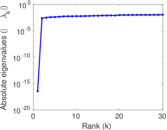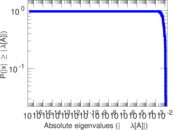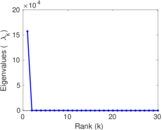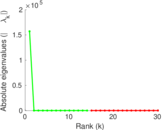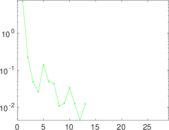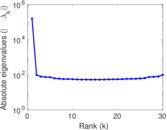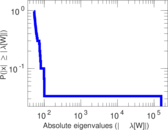# Actors (DBpedia)

This is the bipartite network of movies and the actors that have played in them, from the DBpedia project. The nodes in the network are movies and actors. An edge connects a movie will an actor that has played in it. The dataset corresponds to the <http://dbpedia.org/ontology/starring> relation in DBpedia.

 Code `ST` Internal name `dbpedia-starring` Name Actors (DBpedia) Data source http://wiki.dbpedia.org/Downloads AvailabilityDataset is available for download Consistency checkDataset passed all tests Category Feature network Dataset timestamp 2001 ⋯ 2017 Node meaning Movie, actor Edge meaning Starring Network formatBipartite, undirected Edge typeUnweighted, no multiple edges

## Statistics

 Size n = 157,184 Left size n1 = 76,099 Right size n2 = 81,085 Volume m = 281,396 Wedge count s = 3,240,178 Claw count z = 59,090,368 Cross count x = 1,957,317,775 Square count q = 221,363 4-Tour count T4 = 15,295,024 Maximum degree dmax = 321 Maximum left degree d1max = 65 Maximum right degree d2max = 321 Average degree d = 3.580 47 Average left degree d1 = 3.697 76 Average right degree d2 = 3.470 38 Fill p = 4.560 35 × 10−5 Size of LCC N = 134,015 Diameter δ = 38 50-Percentile effective diameter δ0.5 = 8.669 53 90-Percentile effective diameter δ0.9 = 12.544 4 Median distance δM = 9 Mean distance δm = 9.432 82 Gini coefficient G = 0.437 649 Balanced inequality ratio P = 0.343 072 Left balanced inequality ratio P1 = 0.370 304 Right balanced inequality ratio P2 = 0.258 223 Relative edge distribution entropy Her = 0.955 531 Power law exponent γ = 2.199 29 Tail power law exponent γt = 2.931 00 Tail power law exponent with p γ3 = 2.931 00 p-value p = 0.000 00 Left tail power law exponent with p γ3,1 = 5.521 00 Left p-value p1 = 0.573 000 Right tail power law exponent with p γ3,2 = 3.951 00 Right p-value p2 = 0.794 000 Degree assortativity ρ = −0.076 644 6 Degree assortativity p-value pρ = 0.000 00 Spectral norm α = 24.740 9 Algebraic connectivity a = 0.002 628 42 Spectral separation |λ1[A] / λ2[A]| = 1.244 23 Controllability C = 56,054 Relative controllability Cr = 0.356 616

## Plots

### Fruchterman–Reingold graph drawing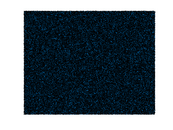### Degree distribution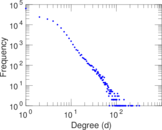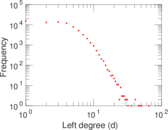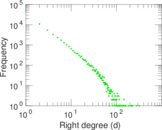### Cumulative degree distribution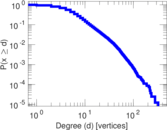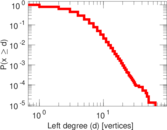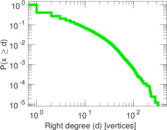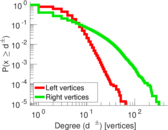### Lorenz curve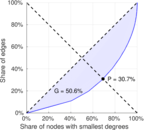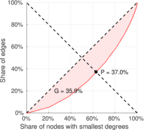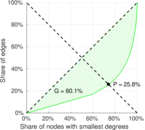### Spectral distribution of the adjacency matrix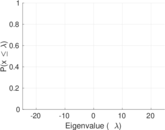### Spectral distribution of the normalized adjacency matrix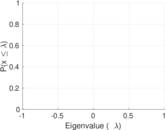### Spectral distribution of the Laplacian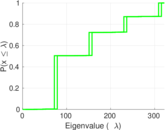### Spectral graph drawing based on the adjacency matrix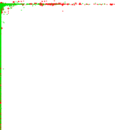### Spectral graph drawing based on the Laplacian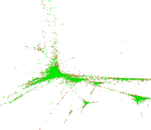### Spectral graph drawing based on the normalized adjacency matrix### Degree assortativity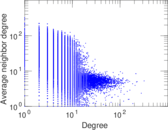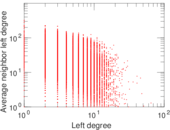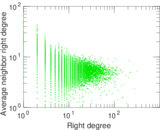### Zipf plot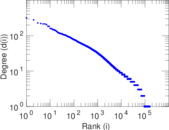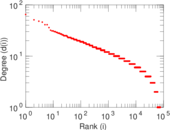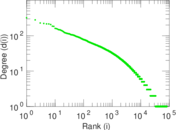### Hop distribution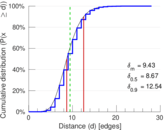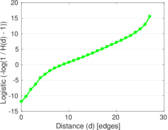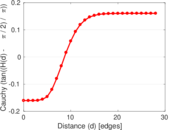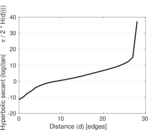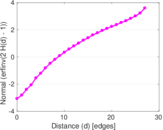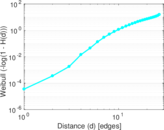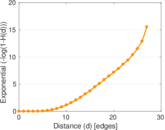### Delaunay graph drawing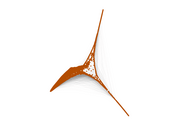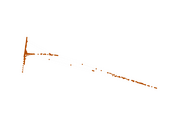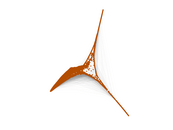### Matrix decompositions plots